## ML Aggarwal Class 8 Solutions for ICSE Maths Chapter 4 Cubes and Cube Roots Ex 4.1

Question 1.
Which of the following numbers are not perfect cubes? Give reasons in support of your answer:
(i) 648
(ii) 729
(iii) 8640
(iv) 8000
Solution:
(i) 648 = 2 × 2 × 2 × 3 × 3 × 3 × 3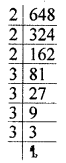Since grouping the prime factors in triplets,
one factor 3 is left on group 1.
So, it is not a perfect cube.

(ii) 729 = 3 × 3 × 3 × 3 × 3 × 3Since grouping the prime factors in triplets,
no factor is left.
So, it is a perfect cube.

(iii) 8640 = 2 × 2 × 2 × 2 × 2 × 2 × 3 × 3 × 3 × 5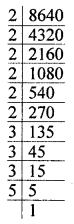Since grouping the prime factors in triplets,
we see that one factor 5 is left without grouping.
So, it is not a perfect cube.

(iv) 8000 = 2 × 2 × 2 × 2 × 2 × 2 × 5 × 5 × 5Since grouping the prime factors in triplets,
we see that no factor is left.
So, it is a perfect cube.

Question 2.
Show that each of the following numbers is a perfect cube. Also, find the number whose cube is the given number:
(i) 1728
(ii) 5832
(iii) 13824
(iv) 35937
Solution:
(i) 1728 = 2 × 2 × 2 × 2 × 2 × 2 × 3 × 3 × 3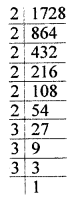Since grouping the prime factors in triplets
we see that no factor is left without grouping.
So, it is a perfect cube, and cube root = 2 × 2 × 3 = 12

(ii) 5832 = 2 × 2 × 2 × 3 × 3 × 3 × 3 × 3 × 3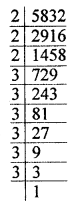Since grouping the prime factors in triplets,
we see that no factor is left without grouping.
So, it is a perfect cube and its cube root is 2 × 3 × 3 = 18

(iii) 13824 = 2 × 2 × 2 × 2 × 2 × 2 × 2 × 2 × 2 × 3 × 3 × 3Since grouping the prime factors in triplets,
no factor is left without grouping.
So, it is a perfect cube and its cube root
= 2 × 2 × 2 × 3
= 24

(iv) 35937 = 3 × 3 × 3 × 11 × 11 × 11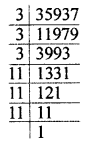Since grouping the prime factors in triplets,
no factor is left without grouping.
So, it is a perfect cube and its cube root is 3 × 11 = 33

Question 3.
Find the smallest number by which each of the following numbers must be multiplied to obtain a perfect cube:
(i) 243
(ii) 3072
(iii) 11979
(iv) 19652
Solution:
(i) 243 = 3 × 3 × 3 × 3 × 3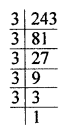Since grouping, the prime factors in triplets 3 × 3 are left.
So, in order to complete in a group of 3, one factor 3 is required.
So, the required smallest number = 3

(ii) 3072 = 2 × 2 × 2 × 2 × 2 × 2 × 2 × 2 × 2 × 2 × 3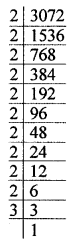Since grouping the prime factors in triplets,
we see that 2 × 3 are left.
So, in order to complete them in a group of
3’s we need 2 × 2 × 3 × 3 to multiply.
∴ The required smallest number = 36

(iii) 11979 = 3 × 3 × 11 × 11 × 11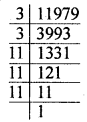Since grouping the prime factors in triplets
3 × 3 are left without grouping in 3’s.
In order to complete it in triplets, one 3 is required.
So, the least number multiply = 3.

(iv) 19652 = 2 × 2 × 17 × 17 × 17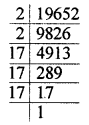Since grouping the prime factors in triplets, 2 × 2 are left.
So, in order to complete it in a triplet,
one 2 is required to multiples.
∴ Required least number to multiply = 2.

Question 4.
Find the smallest number by which each of the following numbers must be divided to obtain a perfect cube:
(i) 1536
(ii) 10985
(iii) 28672
(iv) 13718
Solution:
(i) 1536 = 2 × 2 × 2 × 2 × 2 × 2 × 2 × 2 × 2 × 3Since grouping the prime factors in triplets,
one factor 3 is left without grouping.
So, in order to make it a perfect cube,
it must be divided by 3.
Hence, the required least number = 3

(ii) 10985 = 5 × 13 × 13 × 13Since grouping the prime factors in triplet,
we see that one factor 5 is left without grouping.
So, it must be divided by 5 in order to get a perfect cube.
∴ Required least number = 5

(iii) 28672 = 2 × 2 × 2 × 2 × 2 × 2 × 2 × 2 × 2 × 2 × 2 × 2 × 7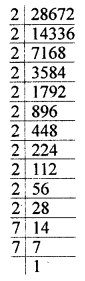Since grouping the prime factors in triplets,
we see that one factor 7 is left without grouping.
So, it must be divided by 7 in order to get a perfect cube.
The required least number = 7

(iv) 13718 = 2 × 19 × 19 × 19Since grouping the prime factors in triplets,
we see that one factor 2 is left without grouping.
So, it must be divided by 2 to get a perfect cube.
∴ The required least number = 2.

Question 5.
Rahul makes a cuboid of plasticine of sides 3 cm × 3 cm × 5 cm. How many such cuboids will he need to form a cube?
Solution:
The given cuboid is 3 cm × 3 cm × 5 cm
To form into a cube, number of such cuboid required
$$\frac{15}{3} \times \frac{15}{3} \times \frac{15}{5}$$
= 5 × 5 × 3 = 75

Question 6.
Find the volume of a cubical box whose surface area is 486 cm2.
Solution:
Surface area of a cubical box = 486 cm2
∴ Side = $$\sqrt{\frac{486}{6}}=\sqrt{81}$$ = 9 cm
Now volume = (Side)3
= (9)3 = 9 × 9 × 9 = 729 cm3

Question 7.
Which of the following are cubes of even natural numbers or odd natural numbers:
(i) 125
(ii) 512
(iii) 1000
(iv) 2197
(v) 4096
(vi) 6859
Solution:
We know that the cube of an even number is even and of an odd number is odd.
So, a cube of 125, 2197 and 6859 are odd and
the cube of 512, 1000 and 4096 are even.

Question 8.
Write the ones digit of the cube of each of the following numbers:
(i) 231
(ii) 358
(iii) 419
(iv) 725
(v) 854
(vi) 987
(vii) 752
(viii) 893
Solution:
We know that cube of number having
1, 4, 5, 6 or 9 in unit place,
then its cube ends in 1, 4, 5, 6 or 9 and if the numbers have
2 in unit place, then cube ends in 8
8 in unit place, then cube ends in 2
3 in unit place then cube ends in 7
7 in unit place then cube ends in 3
and 3 in unit place then cube ends in 7
and 0 in unit place then cube ends in 0, be there will be 3 zeros.
So,
(i) Unit digit of number 231 is 1 then its cube will end in 1.
(ii) Unit digit of number 358 is 8, then its cube will end in 2.
(iii) Unit digit of number 419 is 9, then its cube will end in 9.
(iv) Unit digit of number 725 is 5, then its cube will end in 5.
(v) Unit digit of number 854 is 4, then its cube will end in 4.
(vi) Unit digit of number 987 is 7, then its cube will end in 3.
(vii) Unit digit of number 752 is 2, then its cube will end in 8.
(viii) Unit digit of numbers 893 is 3, then its cube will end in 7.

Question 9.
Find the cubes of the following numbers:
(i) 13
(ii) $$3 \frac{1}{5}$$
(iii) $$-5 \frac{1}{7}$$
Solution:
(i) Cube of-13 = (-13)3
= (-13) × (-13) × (-13) = -2197